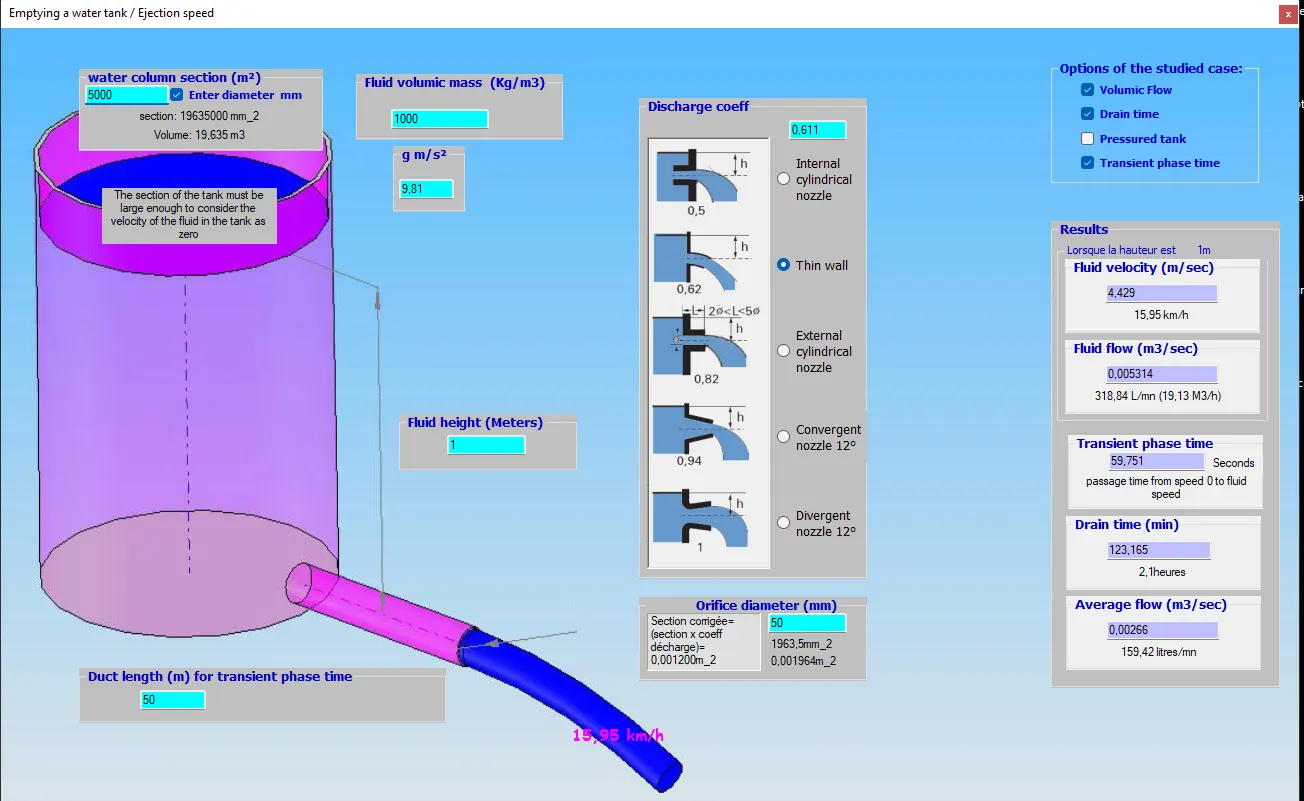Nozzle height / leakage rate

A nozzle is a device that regulates the leakage rate of a liquid or a gas. The presence of a nozzle modifies the flow conditions in a thin wall and produces a certain pressure drop. the flow rate calculation for orifices and nozzles depends on the head, the area of ​​the orifice and a flow coefficient depending on the shape of the nozzle::Relation height and leakage rate according to the shape of the nozzle in the pressure loss calculation software Mecaflux Standard

The fluid speed leaving through the orifice of a reservoir as a function of the height of water, if the reservoir is in the open air, is given by :

Torricelli's formula gives us v = √2g.z

Where

g is gravity(9.81ms²)

z is water height (m)

The emptying time depends on the ratio between the sections of the tank and the orifice and varies over time depending on the height of water remaining in the tank..

The integral formula gives: Drain time= S/s.√2h/g

ou

g is gravity(9.81ms²)

h the water height (m)

S the section of the tank

s the section of the orifice

But the exit of the jet undergoes a disturbance, a pressure drop, when passing the nozzle, which modifies the head or height at the level of the orifice, and thus the speed and therefore the leak rate of the fluid leaving the tank on the real flow with the loss of pressure of the nozzle, is therefore lower than the theoretical flow according to the formula of Torricelli quoted above.

A flow coefficient K (discharge coefficient) is applied which verifies:

outlet speed = K. √(2g.z)

In Mecaflux standard The leakage rate according to the height and the nozzle is calculated using the "Tank emptying" tool where we select the height of the tank, the section of the orifice, and the flow coefficient K given by the shape of the chosen nozzle

The time to empty at the leak rate, from the tank to the open air or under pressure, varies, depending on the shape of the nozzle selected. The average flow, the instantaneous flow, the transient phase time (time that the fluid takes to reach the maximum flow just after the opening of the nozzle orifice) are given:Mecaflux standard software makes it possible to quickly assess the leak rates of various tank heights and nozzle shapes, when the case is a simple tank flowing in the open air through an orifice. The evaluation of the flow of a network of pipes, with ramifications, or singular elements, more complex than a simple tank emptying through a nozzle, requires an approach with evaluations of pressure drops, balancing of branches and other provisions. calculations that come out of this simple case

The flow coefficient of the nozzle is similar to the single head loss coefficient, calculations of the leakage rate of a network of pipes and other singular elements connected to a reservoir, depending on the shape of the reservoir's nozzle, the height of the water intake and the difference in level will be more easily carried out with software integrating a 3D drawing of the network with the elevation heights, and the insertion of singular elements and pipes in this 3D space. The Mecaflux Pro3D software will then be used, see: tank emptying with a gravity flow pipe with Mecaflux Pro3D software.